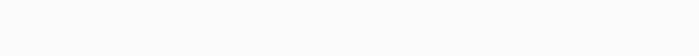# How To Become Masters Of Probability?man studying

Probability in very general terms means the possibility. This is the branch of mathematics that will be dealing with the occurrence of a random event in the whole process and the value can be perfectly expressed from 0-1. Probability has been introduced in the world of mathematics to predict how likely any kind of event will be happening and the basic meaning of the term probability is the expression to which something will likely be happening. This is the basic probability theory that can be perfectly used by the people in the world of probability distribution and with the help of this concept people will be learning the possibility of outcomes for a random experiment as well. To find out the probability of a single event to occur people need to have a clear-cut idea about the total number of possible outcomes as well.

The probability is considered to be the best possible measure of the likelihood of any kind of event to occur and there are several kinds of events that cannot be predicted with total certainty. The probability of all the events into a sample space will always add up to 1.

## The formula for the concept of probability has been explained as follows:

Probability of any kind of event to happen suppose event name is E is equal to the number of favourable outcomes/total number of outcomes

There are several kinds of students who get confused between the concept of desirable outcome and favourable outcome which is the main reason that following the above-mentioned formula is the key to success in this particular area so that they are always able to find out the right answers very accurately.

The probability tree diagram also helps in making sure that organisation, as well as visualisation of the things, will be perfectly undertaken with the help of different possible outcomes. Apart from this the branches and ends of the trees are the two main positions in the whole process. The probability of each branch will be written on the branch and the ends will be containing the outcome. The tree diagram can be perfectly using terms of figuring out when to multiply and went to add. One should also be clear about the concept of a tree diagram for the coin.

## Following are the basic types of probability which the people need to be clear about:

• Theoretical probability: This will always be based on the possible chances of something to happen at this particular probability is mainly based upon the reasoning behind the probability. If a coin has been tossed the theoretical probability of getting the head will be 1/2.
• Experimental probability: This particular probability will always be based upon the observations of a particular experiment and can be calculated depending upon the number of possible outcomes based upon the number of trials. If a particular coin has been tossed 10 times then the head has been recorded six times so the probability of occurring of heads will be 6/10.
• Axiomatic probability: This particular probability will always be based upon a comprehensive set of rules and regulations which have been set by different kinds of people. With the help of this particular approach the chances of occurrence or nonoccurrence can be easily qualified and the axiomatic probability lesson will help in covering the three rules associated with the examples of the Kolmogorov.

Being clear about the concept of complementary events is another very important thing to be taken into consideration by the kids so that they have a clear-cut idea about the whole process and are very much successful in terms of finding the answers to the probability system. The probability theory is considered to be the branch of mathematics that will deal with the possibility of happening of the event and being clear about this particular thing is another very important thing to be undertaken by the kids. Hence, registering the kids on platforms like Cuemath is the best possible way of ensuring that they learn math from the house of experts and can become masters of probability very easily.